Create a matrix showing which planning units are within a certain spatial proximity to each other.

## Usage

proximity_matrix(x, distance)

# S3 method for Raster
proximity_matrix(x, distance)

# S3 method for SpatRaster
proximity_matrix(x, distance)

# S3 method for SpatialPolygons
proximity_matrix(x, distance)

# S3 method for SpatialLines
proximity_matrix(x, distance)

# S3 method for SpatialPoints
proximity_matrix(x, distance)

# S3 method for sf
proximity_matrix(x, distance)

# S3 method for default
proximity_matrix(x, distance)

## Arguments

x

terra::rast() or sf::sf() object representing planning units.

distance

numeric distance threshold. Planning units that are further apart from each other than this threshold are not treated as being within proximity of each other.

## Value

A dsCMatrix symmetric sparse matrix object. Each row and column represents a planning unit. Cells values indicate if the pair-wise distances between different planning units are within the distance threshold or not (using ones and zeros). To reduce computational burden, cells among the matrix diagonal are set to zero. Furthermore, if the argument to x is a terra::rast() object, then cells with missing (NA) values are set to zero too.

## Details

Proximity calculations are performed using sf::st_is_within_distance().

Proximity matrix data might need rescaling to improve optimization performance, see rescale_matrix() to perform these calculations.

## Examples

# \dontrun{
sim_pu_raster <- get_sim_pu_raster()
sim_pu_polygons <- get_sim_pu_polygons()
sim_pu_lines <- get_sim_pu_lines()
sim_pu_points <- get_sim_pu_points()

# create proximity matrix using raster data
## crop raster to 9 cells to provide a small example
r <- terra::crop(sim_pu_raster, c(0, 0.3, 0, 0.3))

## make proximity matrix using a distance threshold of 2
cm_raster <- proximity_matrix(r, distance = 2)

# create proximity matrix using polygon data
## subset 9 polygons to provide a small example
ply <- sim_pu_polygons[c(1:3, 11:13, 20:22), ]

## make proximity matrix using a distance threshold of 2
cm_ply <- proximity_matrix(ply, distance = 2)

# create proximity matrix using line data
## subset 9 lines to provide a small example
lns <- sim_pu_lines[c(1:3, 11:13, 20:22), ]

## make proximity matrix
cm_lns <- proximity_matrix(lns, distance = 2)

## create proximity matrix using point data
## subset 9 points to provide a small example
pts <- sim_pu_points[c(1:3, 11:13, 20:22), ]

# make proximity matrix
cm_pts <- proximity_matrix(pts, distance = 2)

## plot raster and proximity matrix
plot(r, main = "raster", axes = FALSE)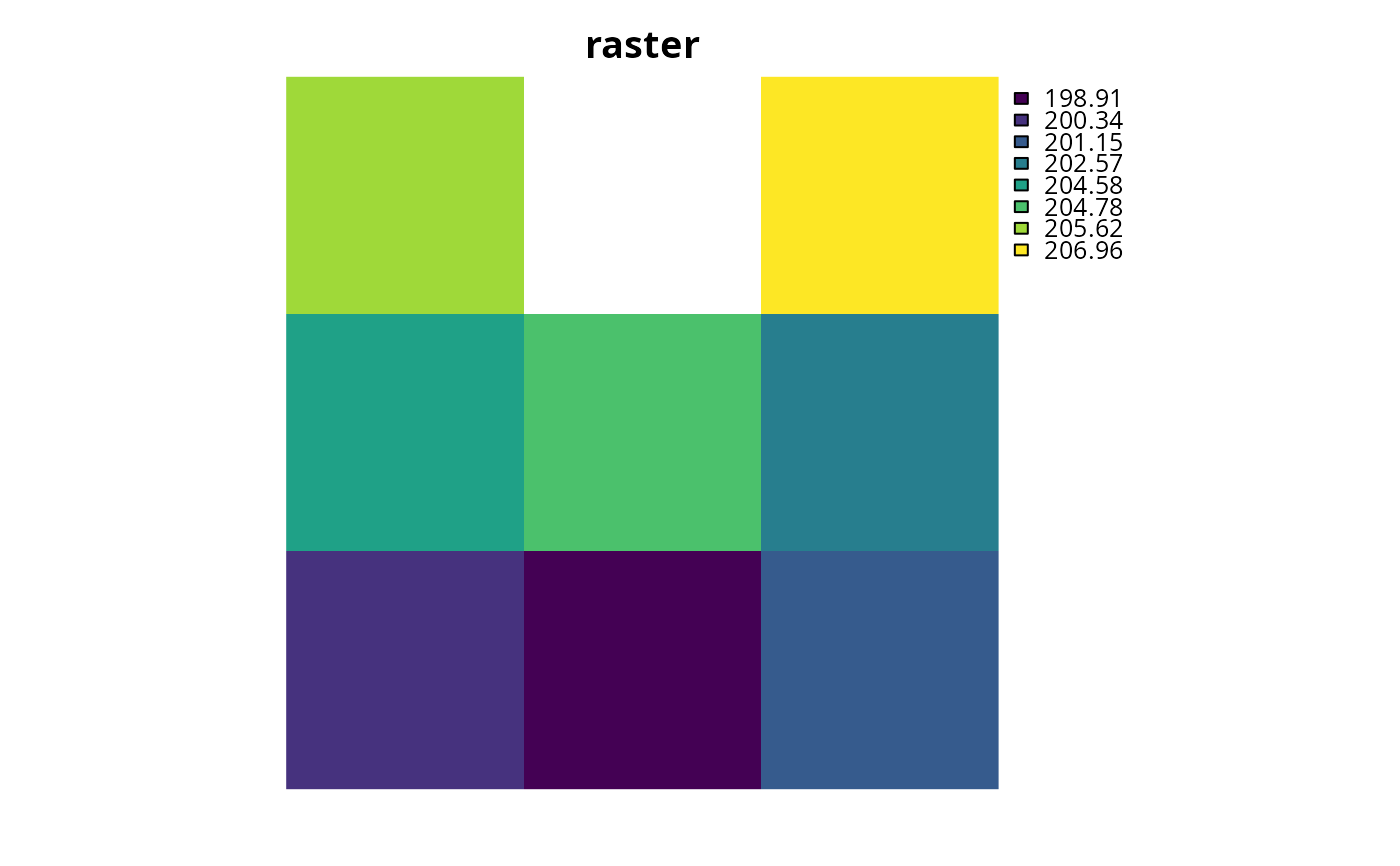Matrix::image(cm_raster, main = "proximity matrix")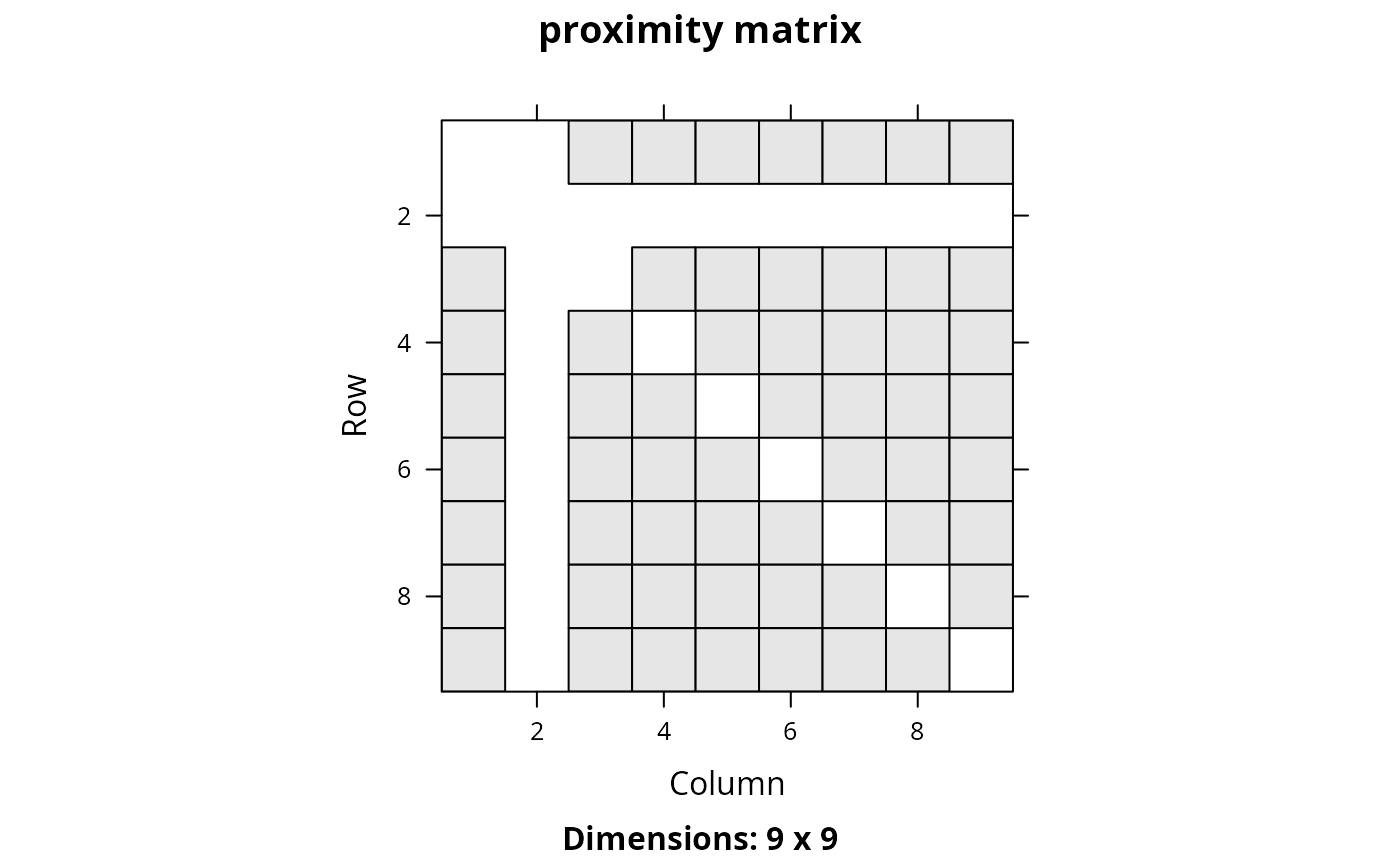## plot polygons and proximity matrix
plot(ply[, 1], main = "polygons", axes = FALSE)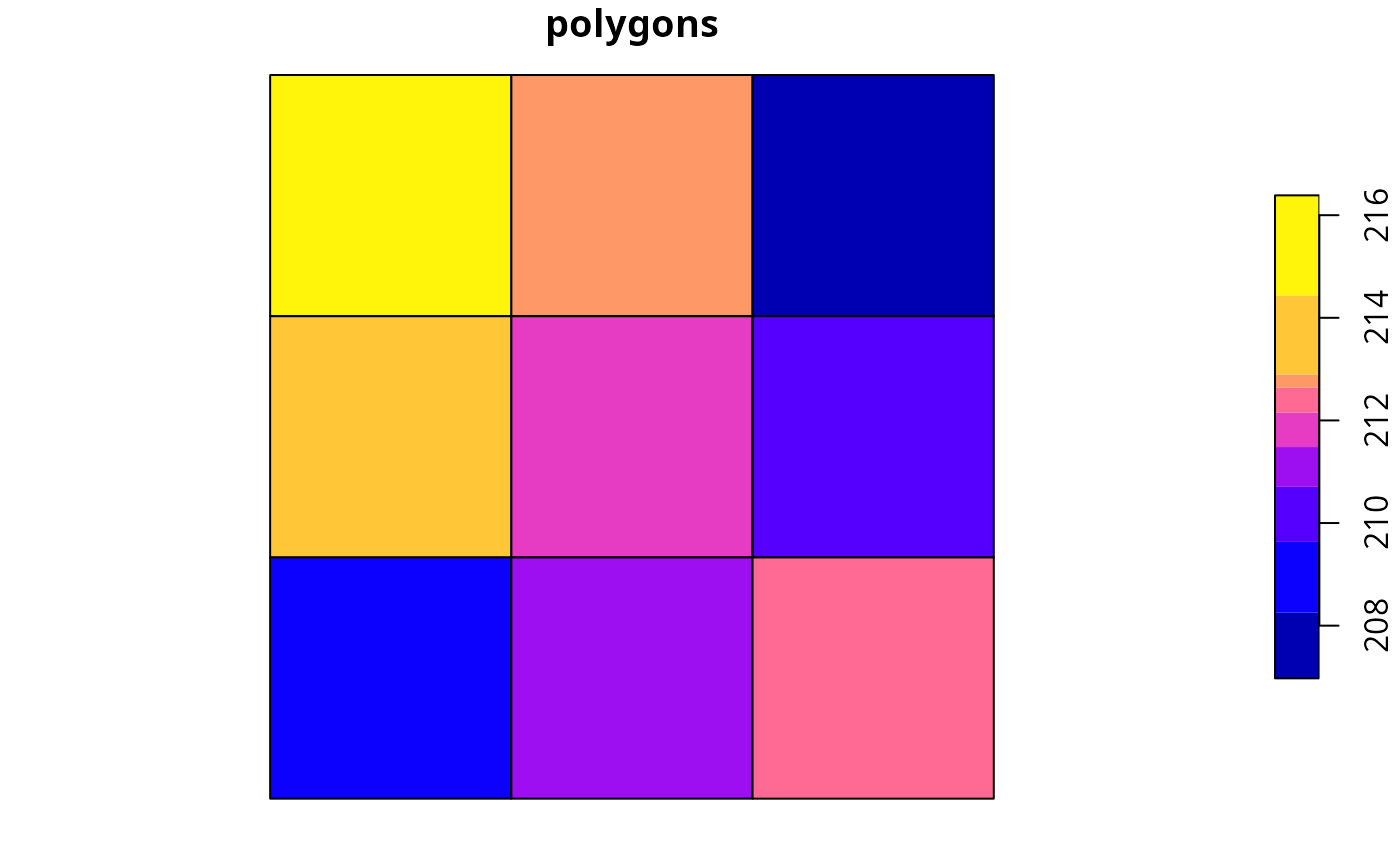Matrix::image(cm_ply, main = "proximity matrix")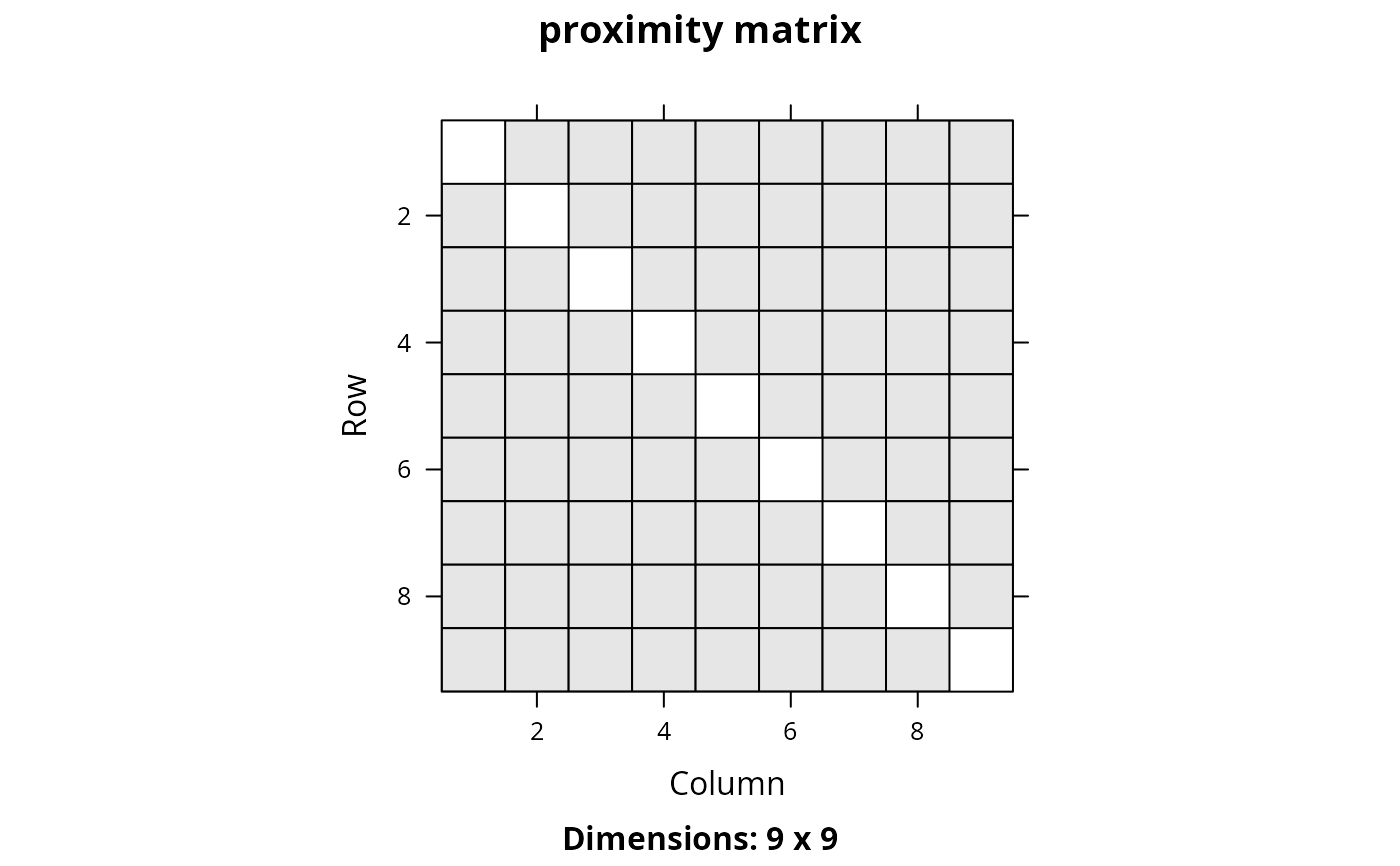## plot lines and proximity matrix
plot(lns[, 1], main = "lines", axes = FALSE)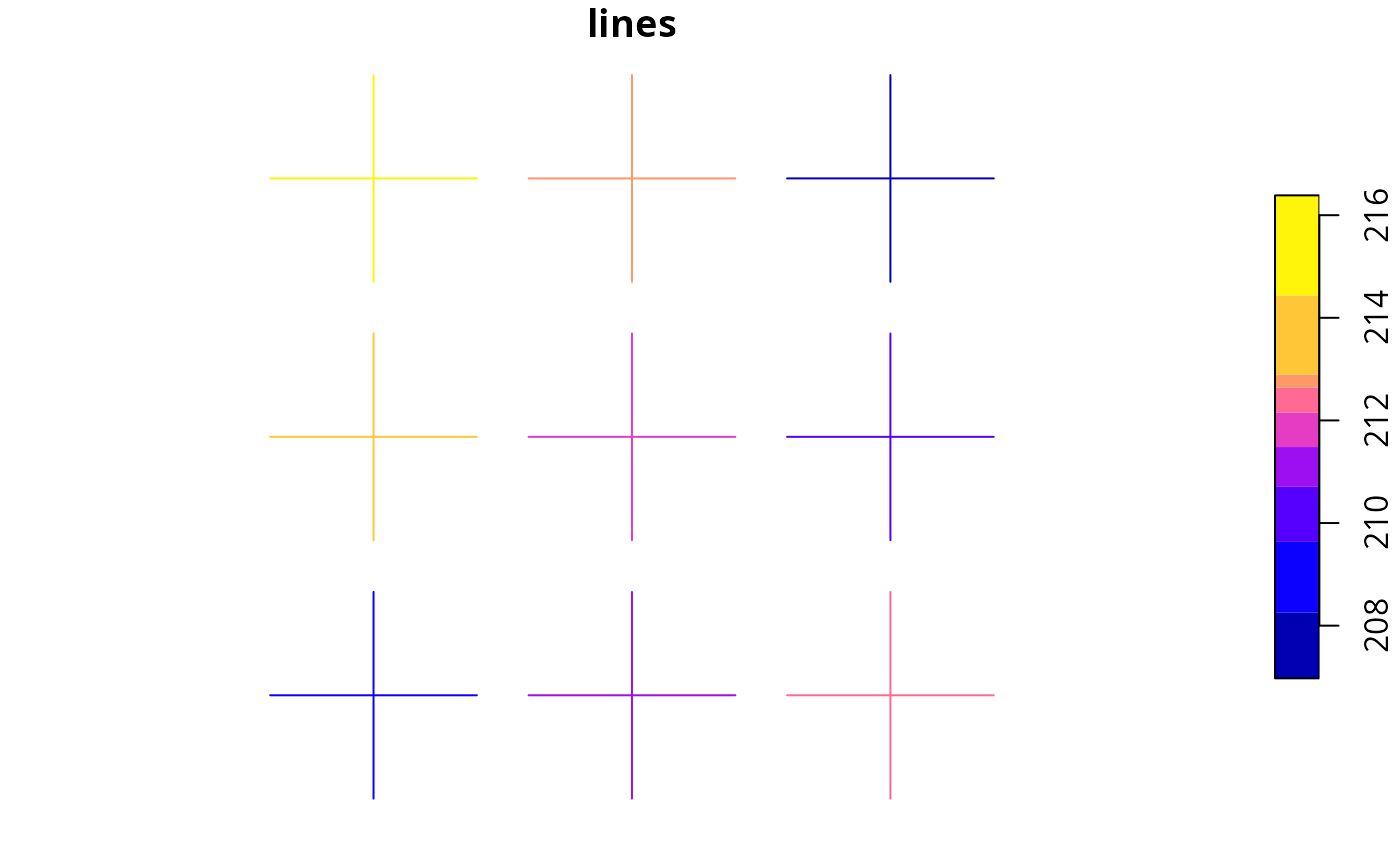Matrix::image(cm_lns, main = "proximity matrix")## plot points and proximity matrix
plot(pts[, 1], main = "points", axes = FALSE)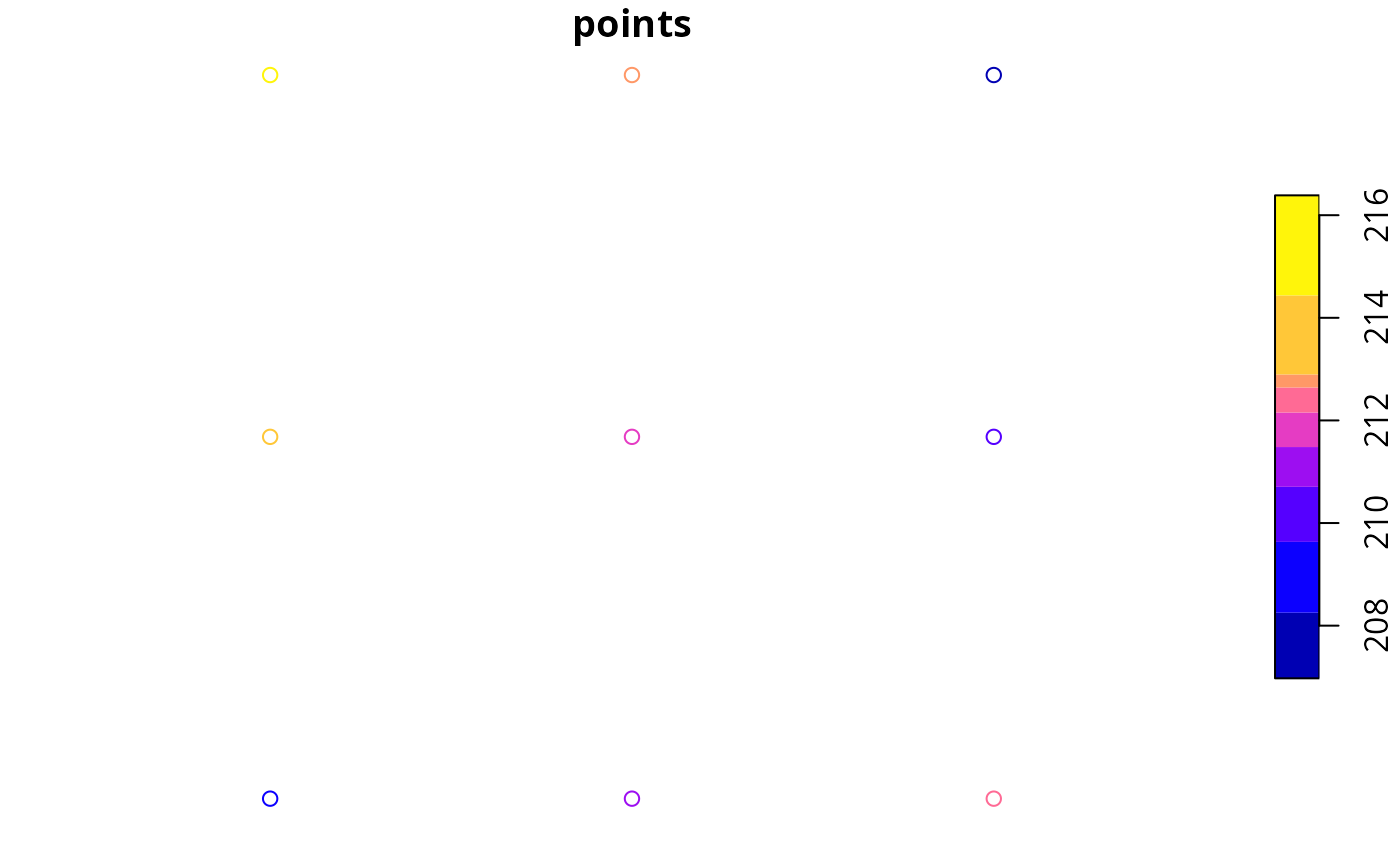Matrix::image(cm_pts, main = "proximity matrix")# }